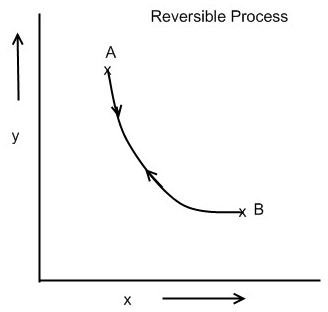# What are Reversible and Irreversible Processes in Thermodynamics?

## Introduction

When the system undergoes a change from its initial state to the final state, the system is said to have undergone a process. During the thermodynamic process, one or more of the properties of the system like temperature, pressure, volume, enthalpy or heat, entropy, etc. changes. The second law of thermodynamics enables us to classify all the processes under two main categories: reversible or ideal processes and irreversible or natural processes.

## What is a Reversible Process?

The process in which the system and surroundings can be restored to the initial state from the final state without producing any changes in the thermodynamics properties of the universe is called a reversible process. In the figure below, let us suppose that the system has undergone a change from state A to state B. If the system can be restored from state B to state A, and there is no change in the universe, then the process is said to be a reversible process. The reversible process can be reversed completely and there is no trace left to show that the system had undergone thermodynamic change.

For the system to undergo reversible change, it should occur infinitely slowly due to infinitesimal gradient. During reversible process all the changes in state that occur in the system are in thermodynamic equilibrium with each other.

Thus there are two important conditions for the reversible process to occur. Firstly, the process should occur in infinitesimally small time and secondly all of the initial and final state of the system should be in equilibrium with each other.

If during the reversible process the heat content of the system remains constant, i.e. it is adiabatic process, then the process is also isentropic process, i.e. the entropy of the system remains constant.

The phenomenon of undergoing reversible change is also called reversibility. In actual practice the reversible process never occurs, thus it is an ideal or hypothetical process.

## Reversible Process## What is an Irreversible Process?

The irreversible process is also called the natural process because all the processes occurring in nature are irreversible processes. The natural process occurs due to the finite gradient between the two states of the system. For instance, heat flow between two bodies occurs due to the temperature gradient between the two bodies; this is in fact the natural flow of heat. Similarly, water flows from high level to low level, current moves from high potential to low potential, etc.

Here are some important points about the irreversible process:

1) In the irreversible process the initial state of the system and surroundings cannot be restored from the final state.

2) During the irreversible process the various states of the system on the path of change from initial state to final state are not in equilibrium with each other.

3) During the irreversible process the entropy of the system increases decisively and it cannot be reduced back to its initial value.

4) The phenomenon of a system undergoing irreversible process is called as irreversibility.

## Reference

Book: Engineering Thermodynamics by P K Nag